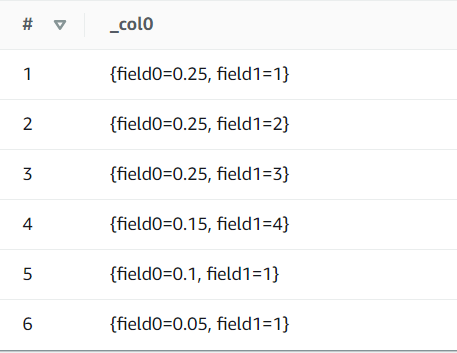# AWS Athena Cookbook

Commonly used snippets for AWS Athena.

Uses the Presto SQL dialect.

# How to insert some data into a table in AWS Athena

Problem You want to insert some data into a table to test something small.

Solution Use the values keyword and name the columns with t(var1, ...).

select *
from
(
values
(-2, .25, 1),
(-3, .25, 2),
(-4, .25, 3),
(-5, .15, 4),
(-6, .10, 1),
(-7, .05, 1)
) as t(offset, weight, weight1)


# What does row() do?

Problem You don’t really understand what row does.

Solution row() combines columns them into a single data object from which you can refer to its fields. In this case the first and second one.

select row(weight, weight2)
from
(
values
(1, .25, 1),
(2, .25, 2),
(3, .25, 3),
(4, .15, 4),
(5, .10, 1),
(6, .05, 1)
) as t(offset, weight, weight2)# How to get the maximum based on another column in AWS Athena

Problem You want to get the value of one column based on the maximum of another column.

Solution Use the max_by(x, y) function that returns the value of x associated with the maximum value of y.

select max_by(value, time)
from
(
values
(1, 5),
(2, 2),
(3, 4)
) as t(time,value)


Returns 4.

# How to get the maximum based on two columns in AWS Athena

Problem You want to get the value of one column based on the maximum of two columns.

Solution Use the max_by(x, y) function that returns the value of x associated with the maximum value of y in combination with row()

select max_by(offset, row(weight1, weight2))
from
(
values
(1, .25, 1),
(2, .25, 2),
(3, .25, 3),
(4, .15, 4),
(5, .10, 1),
(6, .05, 1)
) as t(offset, weight1, weight2)


Returns 3.

# How to get today in AWS Athena

Problem You want to get today’s date in AWS Athena.

Solution Use current_date

select current_date as today-- returns: 2022-06-07


# How to get yesterday in AWS Athena

Problem You want to get yesterday’s date in AWS Athena.

Solution Use current_date together with the interval of one day

select current_date - interval '1' day -- returns: 2022-06-07


Tags:

Categories:

Updated: